Free Printable Worksheets for Fifth Grade

## Algebra: Distribute & Combine Like Terms (Set 1)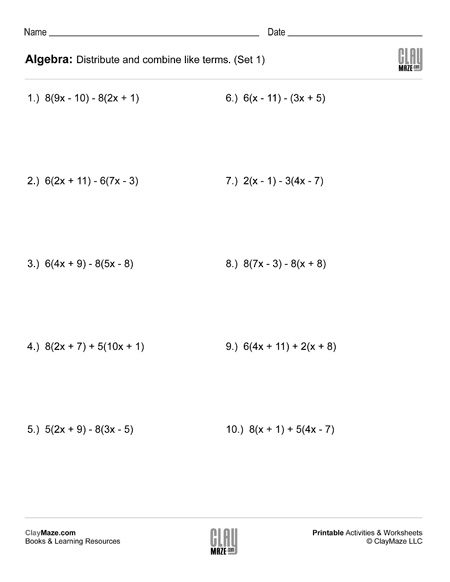Use the worksheet for practice in distributing and combining like terms....

## Algebra: Distribute & Combine Like Terms (Set 2)Algebra worksheet on distributing and combining like terms....

## Algebra: Distribute & Combine Like Terms (Set 3)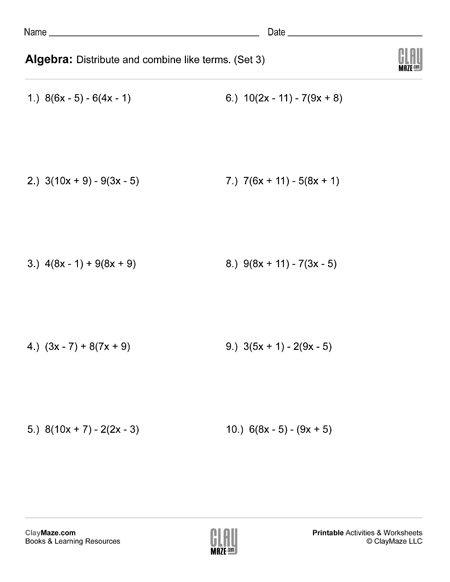Download and print this algebra worksheet on distributing and combining like terms....

## Algebra: Distribute & Combine Like Terms (Set 4)Algebra Worksheet – Distribute and combine the like terms....

## Statistics: Minimum, Maximum, Mean, Median, Mode, Range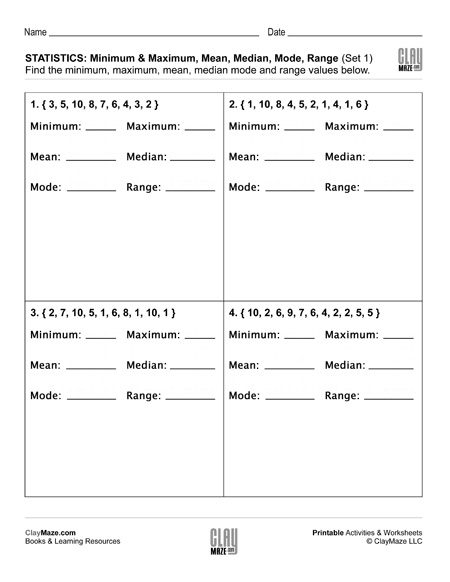Each of the statistics worksheets in this set contains 4 sets of data. The student is asked to find the minimum, maximum, mean, median, mode and range for each data set. These are recommended...

## Basic Statistics: Probability I (Set 1)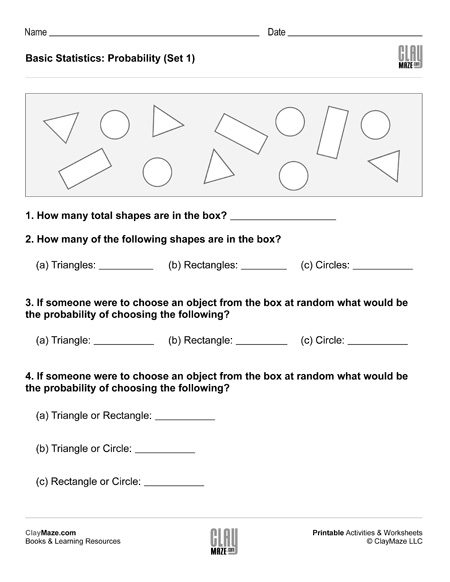This statistics worksheet is on basic probability. There are a number of different shapes in the box and the student is asked to find the probabilities of selecting the different shapes at random....

## Basic Statistics: Probability I (Set 2)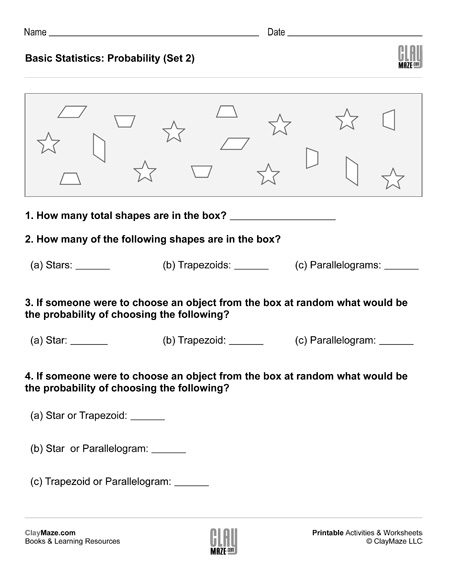This free worksheet covers basic probability. The student is asked several questions about the number of shapes in the box. The student can then use those values to find the probabilities of...

## Basic Statistics: Probability I (Set 3)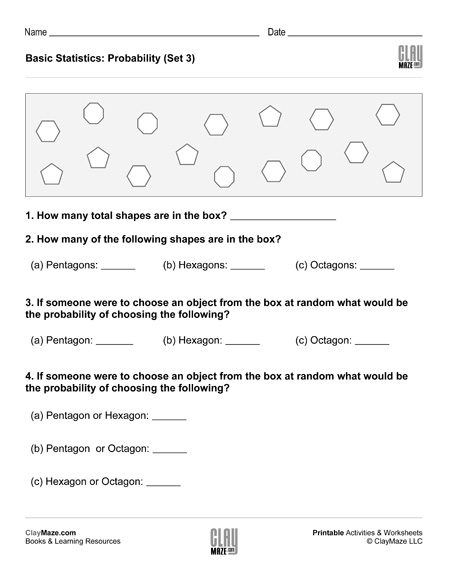This is a basic probability worksheet. The questions are based on the number of shapes in the box and the student is asked to find the probabilities of randomly choosing the different shapes....

## Basic Statistics: Probability I (Set 4)Worksheet on basic probability – The student is asked several questions about the number of shapes in the box and to find the probabilities of selecting different shapes at random....

## Algebra: 1 Step Addition & Subtraction Equations (Set 1)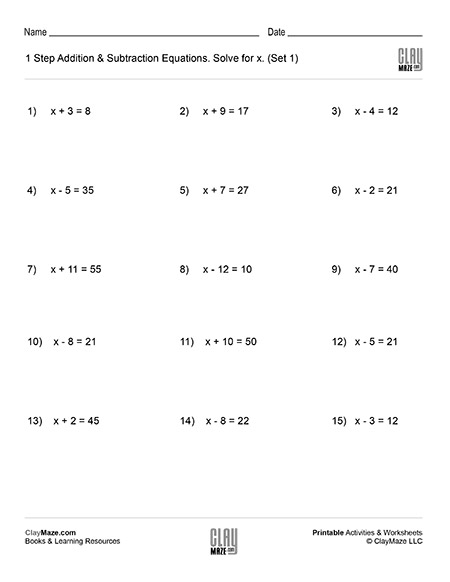This worksheet contains problems that act as an introduction to algebra. The problems are 1 step addition and subtraction equations that ask the student to solve for x. – solutions included...

## Algebra: 1 Step Addition & Subtraction Equations (Set 2)Algebra introduction worksheet. 1 step addition and subtraction equations. – 15 problems with solutions...

## Algebra: 1 Step Addition & Subtraction Equations (Set 3)Intro algebra worksheet: Find the value of x for the problems listed. – 1 step addition and subtraction equations. – with solutions...

## Algebra: 1 Step Addition & Subtraction Equations (Set 4)Free algebra worksheet – 1 step addition and subtraction equations. – 15 problems per page – solutions included...